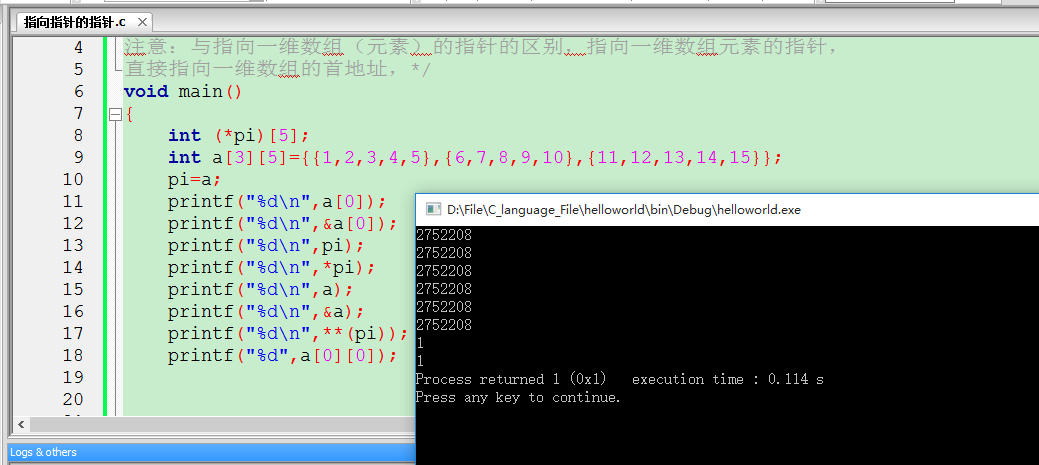• ## 统计-二维随机变量

千次阅读 2018-03-22 20:29:17
二维随机变量
第三章 多维随机变量及其分布

二维随机变量

二维随机变量定义

定义

设E是一个随机试验，它的样本空间是S={e}。设X=X(e)和Y=Y(e)是定义在S上的两个随机变量，它们构成的向量(x,y)称为二维随机变量。

二维随机变量的分布函数定义

设(X,Y)是二维随机变量，对于任意实数x,y,定义二元函数
F(x,y)=P[{X≤x}∩{Y≤y}]=P{X≤x,Y≤y}
F(x,y)称为二维随机变量(x,y)的分布函数

P{x1

二维随机变量的分布函数性质

1. F(x,y)是变量x和y的不减函数，即
x1≤x2=>F(x1,y)≤F（x2,y）
y1≤y2=>F(x,y1)≤F（x,y2）
x1≤x2,y1≤y2=>F(x1,y1)≤F（x2,y2）
F(x,y)朝东北方向上升
F(x,y)朝西南方向下降
因为(x2,y2)左下方的区域较大，随机点落入该区域的概率较大。
2. 0≤F(x,y)≤1 因为概率在这个范围
F(-∞$\infty$,y)=limx→−∞F(x,y)=0$\lim_{x \to -\infty}F(x,y)=0$ 水平朝左走趋于0
F(x,-∞$\infty$)=limy→−∞F(x,y)=0$\lim_{y \to -\infty}F(x,y)=0$ 水平朝下走趋于0
F(-∞$\infty$,-∞$\infty$)=limx→−∞,y→−∞F(x,y)=0$\lim_{x \to -\infty,y \to -\infty}F(x,y)=0$ 水平朝左下走趋于0
F(+∞$\infty$,-+∞$\infty$)=limx→+∞,y→+∞F(x,y)=1$\lim_{x \to +\infty,y \to +\infty}F(x,y)=1$ 水平朝左上走趋于1
3. F(x,y)关于x和y都是右连续
F(x+0,y)=limδx→+0−F(x+δx,y)=F(x,y)$\lim_{\delta x \to +0-}F(x+\delta x,y)=F(x,y)$
F(x,y+0)=limδy→+0+=F(x,y+δy)=F(x,y)$\lim_{\delta y \to +0+}=F(x,y+\delta y)=F(x,y)$
4.若x1

离散型二维随机变量的分布律

定义

如果二维随机变量(X，Y)所有可能的取值是有限对或者可列无限多对，则称(X，Y)是离散型二维随机变量。
设(X，Y)所有可能的取值为(xi,yi),且已知P{X=xi,Y=yi}=pij(i,j=1,2…),则称pij(i,j=1,2…)为二维随机变量(X，Y)的分布律
PX=xi,Y=yj=pij(i,j=1,2...)P{X=x_i,Y=y_j}=p_{ij} (i,j=1,2...)
可以用表格或者矩阵表示

x\y
y1  y2  y3  …

x1
p11 p12 p13 …

x2
p21 p22 p23 …

x3
p31 p32 p33 …

.
.   .   .   …

非负性：pij≥0$p_{ij}≥0$
规范性：∑∞j=1∑∞i=1pij=1$\sum_{j=1}^{\infty}\sum_{i=1}^{\infty}p_{ij}=1$
分布函数：
F(x,y)=P{X≤x,Y≤y}=∑∞xi≤x∑∞yj≤ypij$\sum_{x_i≤x}^{\infty}\sum_{y_j≤y}^{\infty}p_{ij}$

连续型二维随机变量

定义

如果存在非负可积二元函数f(x,y)，使得对应任意实数x,y有
F(x,y)=P{X≤x,Y≤y}∫y−∞∫x−∞f(u,v)dudv$\int_{-\infty}^{y}\int_{-\infty}^{x}f(u,v)dudv$
则称(X,Y)是连续型的二维随机变量，
函数f(x,y)称为(X,Y)的概率密度

性质

1.非负性：F(x,y)≥0
2.规范性：∫+∞−∞∫+∞−∞f(x,y)dxdy=1$\int_{-\infty}^{+\infty}\int_{-\infty}^{+\infty}f(x,y)dxdy=1$
3.设G是xOy坐标面上的一个区域，则点(X,Y)落入G内的概率
P{(X,Y)∈G}\int_\int_{G}f(x,y)dxdy$\int_\int_{G}f(x,y)dxdy$等于曲顶柱体的体积V
4.若f(x,y)在点(x,y)处连续，则在(x,y)处分布律偏导等于概率密度
∂2F∂x∂y=f(x,y)$\frac{\partial^2F}{\partial x\partial y}=f(x,y)$

边缘分布

定义

分布函数F(x,y)=P{X≤x,Y≤y}，而X和Y作为两个随机变量，各自有自己的分布函数，分别记为FX(x)和FY(y):
FX(x)=P{X≤x}
FY(y)=P{Y≤y}
FX(x)和FY(y)分别称为二维随机变量(X,Y)关于X和Y的边缘分布函数

FX(x)=P{X≤x,Y<+∞$\infty$}=F(x,+∞$\infty$)
FY(y)=P{X<+∞$\infty$,Y≤y}=F(+∞$\infty$,y)

离散型二维随机变量的边缘分布

设离散型二维随机变量(X,Y)的概率分布律为
pij=PX=xi,Y=yj(i,j=1,2...)p_{ij}=P{X=x_i,Y=y_j} (i,j=1,2...)
则(X,Y)关于X和Y的边缘分布律分别是：
PX=xi=PX=xi,Y=y1+PX=xi,Y=y2+...=∑∞j=1PX=xi,Y=yj=∑∞j=1Pij=pi·(y任意！)$P{X=x_i}=P{X=x_i,Y=y_1}+P{X=x_i,Y=y_2}+...=\sum_{j=1}^{\infty}P{X=x_i,Y=y_j}=\sum_{j=1}^{\infty}P_{ij}=p_{i·} (y任意！)$
PY=yj=PX=x1,Y=yj+PX=x2,Y=yj+...=∑∞i=1PX=xi,Y=yj=∑∞i=1Pij=p·j(x任意！)$P{Y=y_j}=P{X=x_1,Y=y_j}+P{X=x_2,Y=y_j}+...=\sum_{i=1}^{\infty}P{X=x_i,Y=y_j}=\sum_{i=1}^{\infty}P_{ij}=p_{·j} (x任意！)$

x\y
y1  y2  y3  …

x1
p11 p12 p13 …

x2
p21 p22 p23 …

x3
p31 p32 p33 …

.
.   .   .   …

+
P·1 P·2 P·3

它们在概率分布表边缘，所以叫边缘分布
\sum_{j=1}^{\intfy}\sum_j=1}^{\infty}p_{ij}=1$\sum_{j=1}^{\intfy}\sum_j=1}^{\infty}p_{ij}=1$

连续型二维随机变量边缘分布函数

X的边缘分布函数：
FX(x)$F_X(x)$=P{X≤x,Y<+∞$\infty$}=F(x,+∞$\infty$)
=∫x−∞[∫+∞−∞f(u,y)dy]du$\int_{-\infty}^{x}[\int_{-\infty}^{+\infty}f(u,y)dy]du$
X是连续型随机变量
求导得其概率密度fX(x)=/int+∞−∞f(x,y)dy$f_X(x)=/int_{-\infty}^{+\infty}f(x,y)dy$
Y的边缘分布函数：
FY(y)$F_Y(y)$=P{X<+∞$\infty$,Y≤y}=F(+∞$\infty$,y)
=∫y−∞[∫+∞−∞f(x,y)dx]du$\int_{-\infty}^{y}[\int_{-\infty}^{+\infty}f(x,y)dx]du$
Y是连续型随机变量
求导得其概率密度fY(y)=/int+∞−∞f(x,y)dx$f_Y(y)=/int_{-\infty}^{+\infty}f(x,y)dx$

条件分布

离散型随机变量的条件分布

定义

设(X,Y)是二维离散型随机变量，对于固定的j，若P·j$P_{·j}$=P{Y=yj$y_j$}>0
则称
P{X=xi$x_i$ |Y=yj$y_j$}= {P{X=xi$x_i$，Y=yj$y_j$}} \over {P{Y=yj$y_j$}}= {pij$p_{ij}$} \over {P{·j}}
为在条件Y=yj$y_j$下，随机变量X的条件分布律
反之同理。。。

例子

设二维离散型随机变量(X,Y)的分布律为

x\y
0  1  2

0
0.840 0.060 0.010

1
0.030 0.010 0.005

2
0.020 0.008 0.004

3
0.010 0.002 0.001

1.求边缘分布律
2.求在X=1的条件下，Y的条件分布律
3.求在Y=0的条件下，X的条件分布律

1.

x\y
0  1  2 pi·$p_{i·}$=P{X=xi$x_i$}

0
0.840 0.060 0.010 0.091

1
0.030 0.010 0.005 0.045

2
0.020 0.008 0.004 0.032

3
0.010 0.002 0.001 0.013

p·j$p_{·j}$=P{Y=yj$y_j$}
0.900 0.080 0.020 1

2.

Y=j
0  1  2

P{X=1
Y=j}

3.

X=i
0  1  2 3

P{X=i
Y=o}

连续型随机变量的条件分布

定义

设二维连续型随机变量（X,Y）的概率密度为f(x,y),(X,Y)关于Y的边缘概率密度为fY$f_Y$(y)。若对于固定的y，fY$f_Y$(y)>0,则称f(x,y)/fY$f_Y$(y)为在条件Y=y下，X的条件概率密度，记为
fX|Y(x|y)=f(x,y)fY(y)$f_{X|Y}(x|y)= {f(x,y)} \over {f_Y(y)}$
称∫x−∞fXY(x|y)dx$\int_{-\infty}^xf_{XY}(x|y)dx$为条件Y=y下，X的条件分布函数，记为
FXY(x|y)$F_{XY}(x|y)$=P{X≤x|Y=y}=∫x−∞fXY(x|y)dx$\int_{-\infty}^xf_{XY}(x|y)dx$=1fY(y)∈−∞xf(x,y)dx$1 \over { f_Y(y)} \in{-\infty}^xf(x, y)dx$
反之
Y的条件概率密度
fY|X(y|x)=f(x,y)fX(x)$f_{Y|X}(y|x)= {f(x,y)} \over {f_X(x)}$
称∫y−∞fYX(y|x)dy$\int_{-\infty}^yf_{YX}(y|x)dy$为条件X=z下，Y的条件分布函数，记为
FYX(y|x)$F_{YX}(y|x)$=P{Y≤y|X=x}=∫y−∞fYX(y|x)dy$\int_{-\infty}^yf_{YX}(y|x)dy$=1fX(x)∈−∞xf(x,y)dx$1 \over { f_X(x)} \in{-\infty}^xf(x, y)dx$

例子

相互独立的随机变量

将两个事件的独立性推广到随机变量
两个事件A,B互相独立p(AB)=P(A)P（B）

设二维离散型随机变量(X,Y)的分布律为
Pij$P_{ij}$=P{X=xi$x_i$,Y=yj$y_j$}
则(X,Y)关于X和关于Y的边缘分布律分别是
Pi·$P_{i·}$=P{X=xi$x_i$}=∑∞j=1Pij$\sum_{j=1}^{\infty}P_{ij}$
p·j$p_{·j}$=P{Y=yj$y_j$}=\sum_{i=1}^{\infty}P_{ij}

离散型独立性

定义

设(X,Y)是二维离散型随机变量若
P{X=xi$x_i$,Y=yj$y_j$}=P{X=xi$x_i$}P{Y=yj$y_j$}
即
Pij$P_{ij}$=Pi·p·j$P_{i·}p_{·j}$
则称随机变量X和Y相互独立

例子

随机变量X和Y具有联合分布律

x\y
1  2

0
1/6 1/6

1
2/6 2/6

X和Y是否相互独立？
先求边缘分布律

x\y
1  2 Pi·$P_{i·}$

0
1/6 1/6 1/3

1
2/6 2/6 2/3

P·j$P_{·j}$
1/2 1/2

对于所有i,j均有Pij$P_{ij}$=Pi·p·j$P_{i·}p_{·j}$

连续型对立性

定义

设F(x,y)是二维随机变量(X,Y)的联合分布函数，FX(x)$F_X(x)$和FY(y)$F_Y(y)$分别是(X,Y)关于X和关于Y的边缘分布函数。
若对于任意实数x和y，有
P{X≤x,Y≤y}=P{X≤x}P{Y≤y}
即 F(x,y)=FX(x)$F_X(x)$FY(y)$F_Y(y)$
则称随机变量X和Y相互独立。
联合分布函数等于边缘分布之积

即X和Y相互独立当且仅当它们的联合分布函数等于关于它们的边缘分布函数的乘积。这时，联合分布可由边缘分布唯一确定$\color{red}{联合分布可由边缘分布唯一确定}$。
可以证明：对应连续型二维随机变量(X,Y)X和相互独立当且仅当
f(x,y)=FX(x)$F_X(x)$FY(y)$F_Y(y)$
在平面上几乎处处成立，这时，联合概率密度可由边缘概率密度唯一确定。

在条件Y=y下，X的条件概率密度
fXY(x|y)$f_{XY}(x|y)$ = f(x,y)fY(y)${f(x,y)} \over {f_Y(y)}$ = FX(x)FY(y)fY(y)${F_X(x)F_Y(y)} \over {f_Y(y)}$ =fX(x)$f_X(x)$
同理，在条件X=x下，Y的条件概率密度
f_{YX}(y|y)={f(x,y)} \over {f_X(x)}={$F_X(x)$$F_Y(y)$} \over {f_X(x)}$f_{YX}(y|y)={f(x,y)} \over {f_X(x)}={F_X(x)F_Y(y)} \over {f_X(x)}$ =f_Y(y)
条件概率密度=边缘密度

例子

设二维随机变量(X,Y)的联合密度为
f(x,y)={(1+xy)/4,|x|<1,|y|<10,其他f(x,y)=\left\{
\begin{aligned}
&(1+xy)/4, |x|<1,|y|<1  \\
&0, 其他
\end{aligned}
\right.

问：X与Y是否相互独立

用以下等式验证独立性：
f(x,y)=fX(x)fY(y)
需求边缘概率密度
求x的边缘概率密度
当|x|>1时，f(x,y)=0
fX(x)$f_X(x)$=0
当|x|≤1时，x在-1到1之间
fX(x)$f_X(x)$=∫1−11+xy4dy$\int_{-1}^1 {1+xy} \over {4}dy$=14(∫−11dy+∫1−1xydy)$1 \over 4(\int_-1^1dy+\int_{-1}^1 xydy)$=14$1 \over 4$(1-(-1) + 12$1 \over 2$ - 12)$1 \over 2)$=12$1 \over 2$
∫yxdy$\int_x^ydy$=y22$y^2 \over 2$
得
fX(x)$f_X(x)$=1/2 |x|≤1
类似得y的边缘概率密度
fY(y)$f_Y(y)$=1/2 |x|≤1
当 |x|<1,|y|<1时
f(x,y)=fX(x)fY(y)=1/2 × 1/2 =  1/4≠ 。(1+xy)/4
所以不相互独立

二维正态分布

两个随机变量的函数的分布
展开全文• 用指向一维数组a的指针变量实现对二维数组b中各元素的输入和输出 编写程序，用指向一维数组的指针变量p实现对二维数组b中各元素的输入和输出。要求：输出用指针变量的多种表现形式进行数组元素的多次输出。 完蛋 ...
用指向一维数组a的指针变量实现对二维数组b中各元素的输入和输出
编写程序，用指向一维数组的指针变量p实现对二维数组b中各元素的输入和输出。要求：输出用指针变量的多种表现形式进行数组元素的多次输出。
完蛋 写的时候没发现…现在总感觉我题意理解错了emmmm’
应该没有8哈哈哈
把所有能想到的书上能看到的指针变量的表现形式都写了
奶思！！
#include <stdio.h>

int main(int argc, char const *argv[]) {
int b;
int (*p) = b;
for (int i = 0; i < 6; i++) {
scanf("%d", &p[i]);
}
for (int i = 0; i < 6; i++) {
printf("%d ", (*p)[i]);
}
printf("\n");
for (int i = 0; i < 6; i++) {
printf("%d ", *((*p)+i));
}
printf("\n");
for (int i = 0; i < 2; i++) {
for (int j = 0; j < 3; j++) {
printf("%d ", p[i][j]);
}
}
printf("\n");
for (int i = 0; i < 2; i++) {
for (int j = 0; j < 3; j++) {
printf("%d ", (*(p + i))[j]);
}
}
printf("\n");
for (int i = 0; i < 2; i++) {
for (int j = 0; j < 3; j++) {
printf("%d ", *(p[i] + j));
}
}
printf("\n");
return 0;
}


陕西科技大学 C语言程序设计课作业 指针-20190327  第五题


展开全文C C语言程序设计 数组指针
• 指向一维数组元素的指针变量，指向一维数组的指针变量 指向指针的指针变量（**p）：顾名思义，由于指针运算符的右结合性即 *（*p） 第一个指针只向的是一个地址，然后第个指针指向的才是地址中的值。 指向一维...
指向一维数组元素的指针变量，指向一维数组的指针变量
指向指针的指针变量（**p）：顾名思义，由于指针运算符的右结合性即   *（*p）   第一个指针指向的是一个地址，然后第二个指针指向的才是地址中的值。
指向一维数组的指针变量一般形式：
数据类型  （*指针变量名）[整形常量]
例如：int   (*pi)
以下是我自己的想法（仅供参考）
int a,（*pi）;
pi=a;
指向一维（数组）的指针，指针指向的是一个一维数组，对这个指针取地址    pi    理所应当就是，取一维数组的首地址,注意是地址而不是值，（相当于双重指针第一个指针指向一维数组的首地址），总以为就是对指针变量取值，*pi就是就是指向&a所对应地址的值，但对于指向一维数组的指针确实不是这样的，*p就是二维数组的首地址。
提醒：与指向一维数组（元素）的指针的区别，指向一维数组元素的指针，直接指向一维数组的首地址
如果还感到疑惑就运行下面的代码看看结果，然后在琢磨琢磨，
#include<stdio.h>
void main()
{
int (*pi);             //定义一个指向一维数组的指针
int a={{1,2,3,4,5},{6,7,8,9,10},{11,12,13,14,15}};   //定义一个二维数组
pi=a;                                         //给指针变量赋值
printf("%d\n",a);
printf("%d\n",&a);
printf("%d\n",pi);
printf("%d\n",*pi);
printf("%d\n",a);
printf("%d\n",&a);
printf("%d\n",**(pi));
printf("%d",a);
//其中a和&a和pi和*pi和a和&a，运行的结果相同都是二维数组的首地址

}


运行结果另外:二维数组在内存中的存放顺序为先行后列，可以把二维数组看成一个一维数组（二维数组的每一行看成一个元素），这个一维数组的每个元素有事一个一维数组，
嗯~  就先说这么多吧


展开全文指向指针的指针
• 设EEE是个随机试验，它的样本空间是S=eS={e}S=e,设X=X(e)X=X(e)X=X(e)和Y=Y(e)Y=Y(e)Y=Y(e)是定义在SSS上的随机变量，由它们构成的个向量(X,Y)(X,Y)(X,Y)叫做二维随机向量。 分布函数： 设(X,Y)(X,Y)(X,Y)是...
二维随机变量（向量）
设$E$是一个随机试验，它的样本空间是$S={e}$,设$X=X(e)$和$Y=Y(e)$是定义在$S$上的随机变量，由它们构成的一个向量$(X,Y)$叫做二维随机向量。
分布函数：
设$(X,Y)$是二维随机变量，对于任意实数$x,y$二元函数
$F(x,y)=P\{(X\le x)\cap(Y\le y)\}\overset{记为}{=}P\{X\le x,Y\le y\}$
称为二维随机变量$(X,Y)$的分布函数，或称为随机变量$X$和$Y$的联合分布函数
性质：

$F(x,y)$是变量$x,y$的不减函数
$0\le F(x,y) \le 1$，且

对于任意固定的$y,F(-\infty,y)=0,$
对于任意固定的$x,F(x,-\infty)=0,$
$F(-\infty,-\infty)=0,F(\infty,\infty)=1$

$F(x,y)$关于$x$右连续，关于$y$也右连续
$F(x+0,y)=F(x,y),F(x,y+0)=F(x,y)$
对于任意$(x_1,y_1),(x_2,y_2),x_1<x_2,y_1<y_2$下述不等式成立
$F(x_2,y_2)-F(x_2,y_1)+F(x_1,y_1)-F(x_1,y_2) \ge 0$

离散型随机变量
定义：
如果二维随机变量$(X,Y)$全部可能取到的值是有限对或者可列无限多对，则称$(X,Y)$是离散型随机变量。
联合分布律：
设二维离散随机变量$(X,Y)$所有可能取的值$(x_i,y_j),ij=1,2,...,$记$P\{X=x_i,Y=y_j\}=p_{ij},i,j=1,2,...,$则由概率定义有$p_{ij}\ge 0,\sum_{i=1}^{\infty}\sum_{j=1}^{\infty}p_{ij}=1$
称$(x_i,y_j),i,j=1,2,...,$记$P\{X=x_i,Y=y_j\}=p_{ij},i,j=1,2,...,$为二维离散型随机变量$(X,Y)$的分布律或随机变量$X$和$Y$的联合分布律。
连续型的二维随机变量及其联合概率密度：
如果存在非负的函数$f(x,y)$使对于任意$x,y$有
$F(x,y)=\int_{-\infty}^{y}\int_{-\infty}^{x}f(\mu,\upsilon)d\mu d\upsilon$
则，称$(X,Y)$是连续型的二维随机变量，函数$f(x,y)$称为二维随机变量$(X,Y)$的概率密度，或称随机变量$X$和$Y$的联合概率密度。
性质：

$f(x,y)\ge0$
$\int_{-\infty}^{\infty}\int_{-\infty}^{\infty}f(x,y)dx dy=F(\infty,\infty)=1$
设$G$是$xoy$平面内的区域，点$(X,Y)$落入$G$内的概率为
$P\{(X,Y)\in G\}=\int\int_{G}f(x,y)dxdy$
若$f(x,y)$在点$(x,y)$连续，则有
$\frac{\partial^2F(x,y)}{\partial x\partial y}=f(x,y)$

$n$维随机向量（变量）
定义：
设$E$是一个随机试验，它的样本空间是$S=\{e\}$，设$X_1=X_1\{e\},X_2=X_2\{e\},...,X_n=X_n\{e\}$是定义在$S$上的随机变量，由它们构成一个$n$维向量$(X_1,X_2,...,X_n)$称为$n$维随机向量（变量）
联合分布函数
对于任意$n$个实数$x_1,x_2,..,x_n,n$元函数
$F(x_1,x_2,...,x_n)=P\{X_1\le x_1,X_2\le x_2,...,X_n\le x_n\}$
称为$n$维随机变量的联合分布函数。
ToBeContinued


展开全文• 概率论对于学习 NLP 方向的人，重要性不言而喻。于是我打算从概率论基础篇开始复习，也顺便巩固巩固基础。 这是基础篇的第四篇知识点总结 知识点：二维离散型随机变量 ...NLP基础
• 指向一维数组的指针变量和指向二维数组的指针变量以及指针数组的区别 一、指向一维数组的指针变量 设一维数组为a[n] 定义方法： *指针变量名 即 *P  这个p一般指向的一维数组的首地址，即p=a，...语言
• 1. 二维离散型随机变量的条件分布 2. 二维连续型随机变量的条件分布
• linux 进阶2--C++读取lua文件中的变量一维表、二维
• 概率论基础（3）一维随机变量（离散型和连续型） 概率论基础（4）五种重要的分布（二项、泊松、均匀、指数、正态分布） 概率论基础（5）离散型二维随机变量 连续型二维随机变量 知识点 概率密度 边缘...NLP基础
• 为完成数值分析课的编程作业，自己需要编写个由用户来输入线性方程组系数矩阵以及线性方程组数，然后由程序来给出方程组的解这么个小程序。...全局变量的实现：第步:含有main函数的.c文件中定义int a;qt vector
• 变量的创建 方法 使用方括号“【】”操作符： 例1：创建数组（行向量）a=【1 3 pi 3+5i】 >>a=【1 3 pi 3+5i】 %or a=【1,3,pi,3+5i】 a=1.0000 3.0000 3.1416 3.0000+5.0000i 所有的向量元素...matlab
• C语言：定义指向二维数组的指针变量标签： C语言 二维数组 指针 by 小威威1.引入我们知道，要在函数中处理数组，一般要给函数传递指针。那么，对于一维int数组a，我们可以定义一个int *类型的指针变量p指向该...函数 c语言 指针
• 对已有数据表进行一维二维之间的转化： import pandas as pd # 读入数据： df = pd.read_excel('2dims.xls','Sheet1') df df的结构为： 如上图所示df是一个二维表。 # 将二维数据表转化为一维数据表： ...Python
• 数组用于同种数据类型的存储，常规的数组选取的是块连续内存空间来存储同种类型的数据。 1、 静态数组静态数组是在声明时已经确定子数组大小的数组，即数组元素的个数固定不变。在编译期间在栈中分配好内存的...指针
• C程序设计（第四版） 谭浩强 例8.12 个人设计例 8.12 有个3*4的二维数组，要求用指向元素的指针变量输出二维数组各元素的值。代码块：#include int main() { int a, *p; for (p=*a, printf("Please enter...指针
• ## 二维随机变量函数卷积公式的推导

万次阅读 多人点赞 2016-11-02 00:59:00
二维随机变量函数卷积公式的推导@(概率论)给定Z=g(x,y)Z = g(x,y) 通常需要求FZ(z),fZ(z)F_Z(z),f_Z(z)这里是由两个变元依据关系映射到个变元，因此，求得FZ(z)F_Z(z)后，很容易求得fZ(z)f_Z(z),只是个求导的...
• 使用多种方法实现二维离散随机变量概率分布的计算. 可以用于计算互信息等.accumarray mex
• golang是无法直接在二维数组创建时传变量的 m := 2 n := 2 //var dp [m][n]int 这样会报错提示无法使用变量 var dp [][]int for x := 0; x < m+1; x++ { //循环为一维长度 arr := make([]int, n+1) //创建一个...
• 该思维导图为概率论以为随机变量及其分布的大纲以及基本解题思路，内容较为详细。该思维导图为本人依照张宇闭关修炼2020所制作，希望能帮助大家顺利上岸数学 思维导图
• 常见的二维变量使用的绘图形式：散点图、热力图、堆积柱状图、二维线形图  实际操作来看看，首先读取文件 import pandas as pd import warnings warnings.filterwarnings('ignore') reviews = pd.read_csv(&...
• 一般，如果X，Y是定义在样本空间S上的随机变量，那么（X，Y）称为二维随机变量（或称二维随机向量），类似可以定义n维随机变量。 定义：设二维随机变量(X,Y)所有可能的取值为(xi,yj),(i,j=1,2,3…)；（X，Y）取（xi...
• 第三章 二维随机变量及其分布二维随机变量的联合分布边缘分布 二维随机变量 设 XX, YY 为随机变量，称 (X,Y)(X, Y) 为二维随机变量。 联合分布函数 F(x,y)=P{X⩽x,Y⩽y} F(x, y) = P\{X \leqslant x, Y \...
•C++ 数组长度可变 VS2013
• } 九、数组 一维数组 定义数组 格式： 变量名[数量]; 例如： int count; // 定义一个长度100的类型为int的名称为count的数组 double height; // 定义一个长度20的类型为double的名称为height的数组 注：在...编程语言 c语言 c 程序设计...